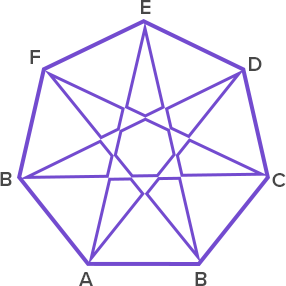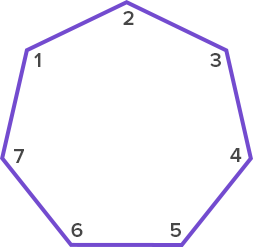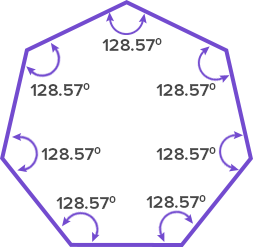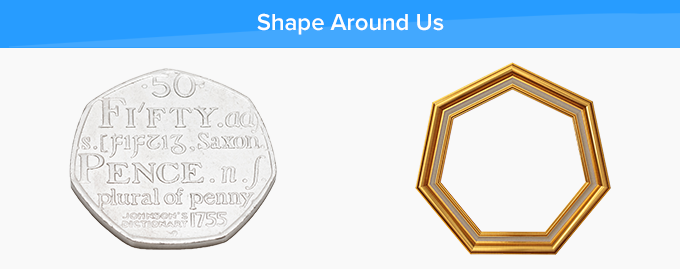# Heptagon - Definition with Examples

The Complete K-5 Math Learning Program Built for Your Child

• 30 Million Kids

Loved by kids and parent worldwide

• 50,000 Schools

Trusted by teachers across schools

• Comprehensive Curriculum

Aligned to Common Core

What is Heptagon?

Heptagon is a polygon ( a closed shape made up of line segments) made up of 7 sides and 7 angles. The word heptagon is made up of two words, hepta meaning seven and gon meaning sides.

There are 14 diagonals in a heptagon and in the given heptagon, the blue line segments represent the diagonals.Some more examples of heptagon are given below:The following figures are not heptagons:There are 8 sides in this figure. So, it is not a heptagon.There are only 5 sides in this figure. So, it is not a heptagon.

Angles of a Heptagon:A heptagon consists of 7 angles, and the sum of these angles is 900°.

∠1 + ∠2 + ∠3 + ∠4 + ∠5 + ∠6 + ∠7 = 900o

 Regular heptagon: Irregular heptagon: If all sides and all angles of a heptagon are equal, then it is known as a regular heptagon. Each angle of the regular heptagon is 128.57 degrees.If the sides and angles of a heptagon are not equal, then it is irregular.Real life examples of the heptagonFun facts about heptagon A heptagon is also known as a septagon. Sept of septagon is a Latin-derived numerical prefix and, hepta is a Greek-derived numerical prefix which means seven and gon means angle.

Won Numerous Awards & Honors